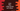# Java program to filter a map by key and value## Java program to filter a map by key and value:

This post will show you how to filter a map by key, value and key-value. We will use a HashMap for the examples. The traditional way is to use a loop to iterate over the map and filter out all the items. These filtered items can be inserted into a new map. Starting from Java 8, we can also use Java Stream filter. This is easy and makes the code concise.

### Example 1: How to use a loop to filter a map:

Let’s use a for loop to filter the content. We will use a HashMap in this example.

``````import java.util.HashMap;
import java.util.Map;

public class Main {
public static void main(String[] args) {
HashMap<Integer, String> numberMap = new HashMap<>();
numberMap.put(0, "Zero");
numberMap.put(1, "One");
numberMap.put(2, "Two");
numberMap.put(3, "Three");
numberMap.put(4, "Four");

HashMap<Integer, String> evenNumberMap = new HashMap<>();
for (Map.Entry<Integer, String> number : numberMap.entrySet()) {
if (number.getKey() % 2 == 0)
evenNumberMap.put(number.getKey(), number.getValue());
}

System.out.println("Filtered even numbers: " + evenNumberMap);
}
}``````
• In this example, we created a HashMap with keys of Integer type and values of String type.
• The for loop is used to filter out all values with even keys. It iterates over the elements of numberMap and filters out all items that have keys with even values.
• The evenNumberMap is another HashMap to hold the output values. We are using the put method to insert the values to this output HashMap.
• At the end of the program, it prints the content of evenNumberMap.

If you run this program, it will print:

``Filtered even numbers: {0=Zero, 2=Two, 4=Four}``

To filter out items based on values, we can use the getValue method. For example,

``````import java.util.HashMap;
import java.util.Map;

public class Main {
public static void main(String[] args) {
HashMap<Integer, String> numberMap = new HashMap<>();
numberMap.put(0, "Zero");
numberMap.put(1, "One");
numberMap.put(2, "Two");
numberMap.put(3, "Three");
numberMap.put(4, "Four");

HashMap<Integer, String> evenNumberMap = new HashMap<>();
for (Map.Entry<Integer, String> number : numberMap.entrySet()) {
if (number.getValue().startsWith("T"))
evenNumberMap.put(number.getKey(), number.getValue());
}

System.out.println("Filtered numbers: " + evenNumberMap);
}
}``````

This is filtering out the values starting with ‘T’.

It will print:

``Filtered numbers: {2=Two, 3=Three}``

### Example 2: Filtering keys of a map with Java Stream API:

We can use Java Stream API to write the above program.

``````import java.util.HashMap;
import java.util.Map;
import java.util.stream.Collectors;

public class Main {
public static void main(String[] args) {
HashMap<Integer, String> numberMap = new HashMap<>();
numberMap.put(0, "Zero");
numberMap.put(1, "One");
numberMap.put(2, "Two");
numberMap.put(3, "Three");
numberMap.put(4, "Four");

Map<Integer, String> evenNumberMap = numberMap
.entrySet()
.stream()
.filter(item -> item.getKey() % 2 == 0)
.collect(Collectors.toMap(Map.Entry::getKey, Map.Entry::getValue));

System.out.println("Filtered even numbers: " + evenNumberMap);
}
}``````
• The stream() method returns a sequential stream of the source HashMap.
• The filter method is used to filter out values from the source stream. It also returns another stream.
• The collect method is used to perform a reduction operation on the stream elements. We are using this method to create a Map with the filtered items.

It will print:

``Filtered even numbers: {0=Zero, 2=Two, 4=Four}``

### Example 3: Filtering values of a map with Java Stream API:

We can use stream with filter() to filter out items based on values.

``````import java.util.HashMap;
import java.util.Map;
import java.util.stream.Collectors;

public class Main {
public static void main(String[] args) {
HashMap<Integer, String> numberMap = new HashMap<>();
numberMap.put(0, "Zero");
numberMap.put(1, "One");
numberMap.put(2, "Two");
numberMap.put(3, "Three");
numberMap.put(4, "Four");

Map<Integer, String> evenNumberMap = numberMap
.entrySet()
.stream()
.filter(item -> item.getValue().startsWith("T"))
.collect(Collectors.toMap(Map.Entry::getKey, Map.Entry::getValue));

System.out.println("Filtered numbers: " + evenNumberMap);
}
}``````

In this example, we are filtering the items with values starting with ‘T’.

``Filtered numbers: {2=Two, 3=Three}``

### Example 4: Filtering keys and values of a map with Java Stream class:

We can use multiple filter() methods. Since the filter method returns a Stream, we can use the filter() method again on the returned stream.

``````import java.util.HashMap;
import java.util.Map;
import java.util.stream.Collectors;

public class Main {
public static void main(String[] args) {
HashMap<Integer, String> numberMap = new HashMap<>();
numberMap.put(0, "Zero");
numberMap.put(1, "One");
numberMap.put(2, "Two");
numberMap.put(3, "Three");
numberMap.put(4, "Four");

Map<Integer, String> evenNumberMap = numberMap
.entrySet()
.stream()
.filter(item -> item.getValue().startsWith("T"))
.filter(item -> item.getKey() % 2 == 0)
.collect(Collectors.toMap(Map.Entry::getKey, Map.Entry::getValue));

System.out.println("Filtered even numbers: " + evenNumberMap);
}
}``````

It will print:

``Filtered numbers: {2=Two}``

We can also combine these two filter() methods:

``````import java.util.HashMap;
import java.util.Map;
import java.util.stream.Collectors;

public class Main {
public static void main(String[] args) {
HashMap<Integer, String> numberMap = new HashMap<>();
numberMap.put(0, "Zero");
numberMap.put(1, "One");
numberMap.put(2, "Two");
numberMap.put(3, "Three");
numberMap.put(4, "Four");

Map<Integer, String> evenNumberMap = numberMap
.entrySet()
.stream()
.filter(item -> item.getValue().startsWith("T") && item.getKey() % 2 == 0)
.collect(Collectors.toMap(Map.Entry::getKey, Map.Entry::getValue));

System.out.println("Filtered numbers: " + evenNumberMap);
}
}``````

It will print the same output.